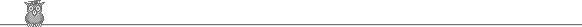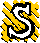35th IMO 1994 shortlisted problemsAlgebra

A1.  The sequence x0, x1, x2, ... is defined by x0 = 1994, xn+1 = xn2/(xn + 1). Show that [xn ] = 1994 - n for 0 ≤ n ≤ 998.A4.  h and k are reals. Find all real-valued functions f defined on the positive reals such that f(x) f(y) = yh f(x/2) + xk f(y/2) for all x, y.A5.  Let f(x) = (x2 + 1)/(2x) for x non-zero. Define f0(x) = x and fn+1(x) = f( fn(x) ). Show that for x not -1, 0 or 1 we have fn(x)/fn+1(x) = 1 + 1/f(y), where y = (x+1)N/(x-1)N and N = 2n.Combinatorics

C1.  Two players play alternately on a 5 x 5 board. The first player always enters a 1 into an empty square and the second player always enters a 0 into an empty square. When the board is full, the sum of the numbers in each of the nine 3 x 3 squares is calculated and the first player's score is the largest such sum. What is the largest score the first player can make (if the second player plays so as to minimise this score)?C2.  A finite graph is connected. A positive real number is assigned to each point. Each point is colored red or blue and at least one point is colored red. Show that, given a knowledge of (1) the points and edges of the graph, (2) the number assigned to each red point, and (3) for each blue point the average of the numbers for the points joined to it, one can find the number assigned to each point.C3.  Peter has three accounts at a bank, each with an integral number of dollars. He is only allowed to transfer money from one account to another if the effect of the transfer is to double the amount of money in one of the accounts. Show that by a series of transfers he can always empty one account, but that he cannot always get all his money into one account.C4.  There are n+1 cells in a row labeled from 0 to n and n+1 cards labeled from 0 to n. The cards are arbitrarily placed in the cells, one per cell. The objective is to get card i into cell i for each i. The allowed move is to find the smallest h such that cell h has a card with a label k > h, pick up that card, slide the cards in cells h+1, h+2, ... , k one cell to the left and to place card k in cell k. Show that at most 2n - 1 moves are required to get every card into the correct cell and that there is a unique starting position which requires 2n - 1 moves. [For example, if n = 2 and the initial position is 210, then we get 102, then 012, a total of 2 moves.]C5.  1994 girls are seated at a round table. Initially one girl holds n tokens. Each turn a girl who is holding more than one token passes one token to each of her neighbours. Show that if n < 1994, the game must terminate. Show that if n = 1994 it cannot terminate. [Note: Sweden proposed the problem with 1991 girls (in which case you must show the game terminates for n ≤ 1991), but the PIG (see below) decided that was too difficult.]C6.  Two players play alternatively on an infinite square grid. The first player puts a X in an empty cell and the second player puts a O in an empty cell. The first player wins if he gets 11 adjacent Xs in a line, horizontally, vertically or diagonally. Show that the second player can always prevent the first player from winning.C7.  n > 2. Show that there is a set of 2n-1 points in the plane, no three collinear such that no 2n form a convex 2n-gon.Geometry

G1.  C and D are points on a semicircle. The tangent at C meets the extended diameter of the semicircle at B, and the tangent at D meets it at A, so that A and B are on opposite sides of the center. The lines AC and BD meet at E. F is the foot of the perpendicular from E to AB. Show that EF bisects angle CFD.G2.  ABCD is a quadrilateral with BC parallel to AD. M is the midpoint of CD, P is the midpoint of MA and Q is the midpoint of MB. The lines DP and CQ meet at N. Prove that N is inside the quadrilateral ABCD.G3.  A circle C has two parallel tangents L' and L". A circle C' touches L' at A and C at X. A circle C" touches L" at B, C at Y and C' at Z. The lines AY and BX meet at Q. Show that Q is the circumcenter of XYZ.G5.  L is a line not meeting a circle center O. E is the foot of the perpendicular from O to L and M is a variable point on L (not E). The tangents from O to the circle meet it at A and B. The feet of the perpendiculars from E to MA and MB are C and D respectively. The lines CD and OE meet at F. Show that F is fixed.Number theory

N1.  Find the largest possible subset of {1, 2, ... , 15} such that the product of any three distinct elements of the subset is not a square.N4.  Define the sequences an, bn, cn as follows. a0 = k, b0 = 4, c0 = 1. If an is even then an+1 = an/2, bn+1 = 2bn, cn+1 = cn. If an is odd, then an+1 = an - bn/2 - cn, bn+1 = bn, cn+1 = bn + cn. Find the number of positive integers k < 1995 such that some an = 0.N6.  Define the sequence a1, a2, a3, ... as follows. a1 and a2 are coprime positive integers and an+2 = an+1an + 1. Show that for every m > 1 there is an n > m such that amm divides ann. Is it true that a1 must divide ann for some n > 1?N7.  A wobbly number is a positive integer whose digits are alternately zero and non-zero with the last digit non-zero (for example, 201). Find all positive integers which do not divide any wobbly numbers.Note: problems A2, A3, G4, N2, N3, and N5 were used in the Olympiad and are not shown here.
Apparently the problems committee for this year was known as the "Problem Interpolation Group (P.I.G.)" ! The previous year it seems to have been simply the Problems Committee, and in 1995 it became the Problems Selection Committee.Shortlist home